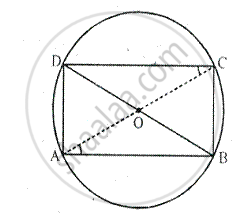Share

# If Two Sides of a Cyclic Quadrilateral Are Parallel; Prove That: (I) Its Other Two Sides Are Equal. (Ii) Its Diagonals Are Equal. - Mathematics

Course

#### Question

If two sides of a cyclic quadrilateral are parallel; prove that:
(i) its other two sides are equal.
(ii) its diagonals are equal.

#### SolutionGiven –
ABCD is a cyclic quadrilateral in which AB ∥ DC. AC and BD are its diagonals.
To prove –
(ii) AC = BD
Proof –

(i) AB || DC ⇒ ∠DCA = ∠CAB           [Alternate angles]

Now, chord AD subtends ∠DCA and chord BC subtends ∠CAB
At the circumference of the circle.

∴ ∠DCA = ∠CAB        [proved]

(ii) Now in ∠ABC and ∠ADB ,
AB = AB               [Common]

∠ACB = ∠ADB     [Angles in the same segment]

By Side – Angle – Side criterion of congruence, we have

The corresponding parts of the congruent triangles are congruent.

∴  AC = BD   [c.p.c.t]

Is there an error in this question or solution?